Thermodynamics MCQ Level - 1

# Thermodynamics MCQ Level - 1

Test Description

## 30 Questions MCQ Test | Thermodynamics MCQ Level - 1

Thermodynamics MCQ Level - 1 for Class 11 2022 is part of Class 11 preparation. The Thermodynamics MCQ Level - 1 questions and answers have been prepared according to the Class 11 exam syllabus.The Thermodynamics MCQ Level - 1 MCQs are made for Class 11 2022 Exam. Find important definitions, questions, notes, meanings, examples, exercises, MCQs and online tests for Thermodynamics MCQ Level - 1 below.
Solutions of Thermodynamics MCQ Level - 1 questions in English are available as part of our course for Class 11 & Thermodynamics MCQ Level - 1 solutions in Hindi for Class 11 course. Download more important topics, notes, lectures and mock test series for Class 11 Exam by signing up for free. Attempt Thermodynamics MCQ Level - 1 | 30 questions in 30 minutes | Mock test for Class 11 preparation | Free important questions MCQ to study for Class 11 Exam | Download free PDF with solutions
 1 Crore+ students have signed up on EduRev. Have you?
Thermodynamics MCQ Level - 1 - Question 1

### An ideal gas is taken around ABCA as shown in theabove P-V diagram. The work done during a cycle is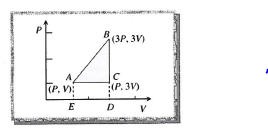Thermodynamics MCQ Level - 1 - Question 2

### If R = universal gas constant, the amount of heat needed to raise the temperature of 2 mol of an idealmonatomic gas from 273 K to 373 K when no work isdone is

Thermodynamics MCQ Level - 1 - Question 3

### During an adiabatic process, the pressure of a gasis found to be proportional to the cube of its absolutetemperature. The ratio CP I CV for the gas is

Thermodynamics MCQ Level - 1 - Question 4

A thermally insulated container is divided into twoparts by a screen. In one part the pressure andtemperature are P and T for an ideal gas filled. In thesecond part it is vacuum. If now a small hole iscreated in the screen, then the temperature of thegas will

Thermodynamics MCQ Level - 1 - Question 5

Two samples A and B of a gas initially at the samepressure and temperature are compressed from volume V to V/2 (A isothermally and B adiabatically).The final pressure of A is

Thermodynamics MCQ Level - 1 - Question 6

In the following indicator diagram, the net amount ofwork done will be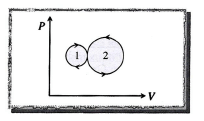Thermodynamics MCQ Level - 1 - Question 7

A cyclic process for 1 mole of an ideal gas is shown inFig. in the V-T, diagram. The work done in AB,BC andCA, respectively, is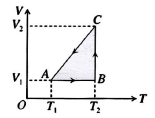Thermodynamics MCQ Level - 1 - Question 8

A cyclic process ABCD is shown in the following P-Vdiagram. Which of the following curves representsthe same process?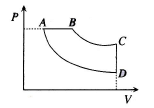Thermodynamics MCQ Level - 1 - Question 9

An ideal gas expands in such a manner that itspressure and volume can be related by equationPV2 = constant. During this process, the gas is

Thermodynamics MCQ Level - 1 - Question 10

A cyclic process ABCA is shown in the V-T diagram. Process on the P-V diagram is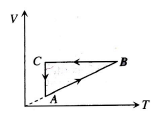Thermodynamics MCQ Level - 1 - Question 11

One mole of a diatomic gas undergoes a processP = P0/[1+(V/V0)3] where P0 and V0 are constants.The translational kinetics energy of the gas whenV = V0 is given by

Thermodynamics MCQ Level - 1 - Question 12

In the given elliptical P-V diagram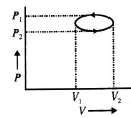Thermodynamics MCQ Level - 1 - Question 13

Three processes compose a thermodynamic cycleshown in the accompanying P-V diagram of an ideal gas.Process1→2 takes place at constant temperature,during this process 60 J of heat enters the system.Process 2→3 takes place at constant volume.During this process 40 J of heat leaves the system.Process3→1 is adiabaticWhat is the change in internal energy of the systemduring process3→1 ?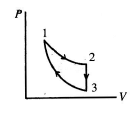Thermodynamics MCQ Level - 1 - Question 14

A sample of an ideal gas is taken through the cyclicprocess ABCA shown in Fig. It rejects 50 J of heatduring the part AB, does not absorb or reject theheat during BC, and accepts 70 J of heat during CA.Forty joules of work is done on the gas during thepart BC. The internal energies at B and C,respectively, will be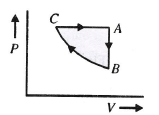Thermodynamics MCQ Level - 1 - Question 15

A certain ideal gas undergoes a polytropic processPVn = constant such that the molar specific heat during the process is negative. If the ratio of the specific heats of the gas be γ , then the range ofvolume of n will be

Thermodynamics MCQ Level - 1 - Question 16

Figure shown an isochore, an isotherm, an adiabaticand two isobars of two gases n a work done versusheat supplied curve. The initial states of both gasesare the same and the scales for the two axes aresame.Which of the following statements is incorrect?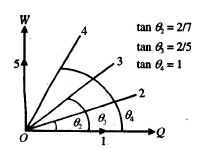Thermodynamics MCQ Level - 1 - Question 17

A stationary cylinder of oxygen used in a hospitalhas the following characteristics at room temperature300 K, gauge pressure 1.38 × 107 Pa, volume 16L.If the flow area, measured at atmospheric pressure,is constant at 2.4 L/min, the cylinder will last for nearly.

Thermodynamics MCQ Level - 1 - Question 18

A thermodynamic process is shown in Fig. Thepressures and volumes corresponding to some pointsin the figure are PA = 3 × 104 Pa VA = 2 × 10-3 mPB = 8 × 104 Pa VD = 5 × 10-3 m3 In the process AB, 600 J of heat is added to thesystem. The change in internal energy of the systemin the process AB would be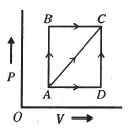Thermodynamics MCQ Level - 1 - Question 19

Carbon monoxide is carried around a closed cyclicprocess abc, in which bc is an isothermal process,as shown in Fig. The gas absorbs 7000 J of heat asits temperature is increased from 300 K to 1000 K ingoing from a to b. The quantity of heat ejected by thegas during the process ca is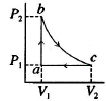Thermodynamics MCQ Level - 1 - Question 20

Pressure versus temperature graph of an ideal gasis an shown in Fig.Corresponding density (ρ) versus volume (V) graphwill be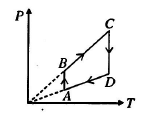Thermodynamics MCQ Level - 1 - Question 21

An ideal gas is taken through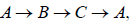asshown in Fig. If the net heat supplied to the gas inthe cycle is 55 J, the work done by the gas in theprocess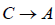is.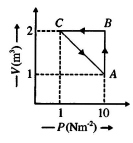Thermodynamics MCQ Level - 1 - Question 22

Two identical containers A and B have frictionlesspistons. They contain the same volume of an idealgas at the same temperature. The mass of the gasin A is mA and that in B is mB. The gas in each cylinderis now allowed to expand isothermally to double theinitial volume. The change in the pressure in A and B, respectively, is Δp and 1.5 Δp , Then

Thermodynamics MCQ Level - 1 - Question 23

Argon gas is adiabatically compressed to half itsvolume. If P,V and T represent the pressure, volumeand temperature of the gaseous system, respectively,at any stage, then the correct equation representingthe process is

Thermodynamics MCQ Level - 1 - Question 24

If the ratio of specific heat of a gas at constantpressure to that at constant volume is g , the changein internal energy of the mass of gas, when thevolume changes from V to 2V at constant pressure P, is

Thermodynamics MCQ Level - 1 - Question 25

A gas is at 1 atm pressure with a volume 800 cm3.When 100 J of heat is supplied to the gas, it expandsto 1 L at constant pressure. The change in its internalenergy is

Thermodynamics MCQ Level - 1 - Question 26

A fixed mass of a gas is first heated isobarically todouble the voule and then cooled isochorically todecrease the temperature back to the initial value.By what factor would the work done by the gasdecreased, had the process been isothermal?

Thermodynamics MCQ Level - 1 - Question 27

An ideal heat engine has an efficiency η . The ciefficient of performance of the engine when driven backward will be

Thermodynamics MCQ Level - 1 - Question 28

The equation of state for a gas is given by PV =ηRT + αV , whereh is the number of molesand a a positive constant. The initial pressure andtemperature of 1 mol of the gas contained in a cylinderis P0 and T0, respectively. The work done by the gaswhen its temperature doubles isobarically will be

Thermodynamics MCQ Level - 1 - Question 29

A container of volume 1 m3 is divided into two equalcompartments by a partition. One of thesecompartments contains an ideal gas at 300 K. Theother compartment is vacuum. The whole system isthermally isolated from its surroundings. The partitionis removed and the gas expands to occupy the wholevolume of the container. Its temperature now would be

Thermodynamics MCQ Level - 1 - Question 30

If one mole of a monoatomic gas γ = 5/3 is is mixed with one mole of a diatomic gas γ = 7/5. The value of V for the mixture is :

 Use Code STAYHOME200 and get INR 200 additional OFF Use Coupon Code
Information about Thermodynamics MCQ Level - 1 Page
In this test you can find the Exam questions for Thermodynamics MCQ Level - 1 solved & explained in the simplest way possible. Besides giving Questions and answers for Thermodynamics MCQ Level - 1, EduRev gives you an ample number of Online tests for practice